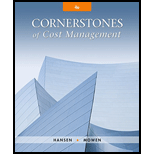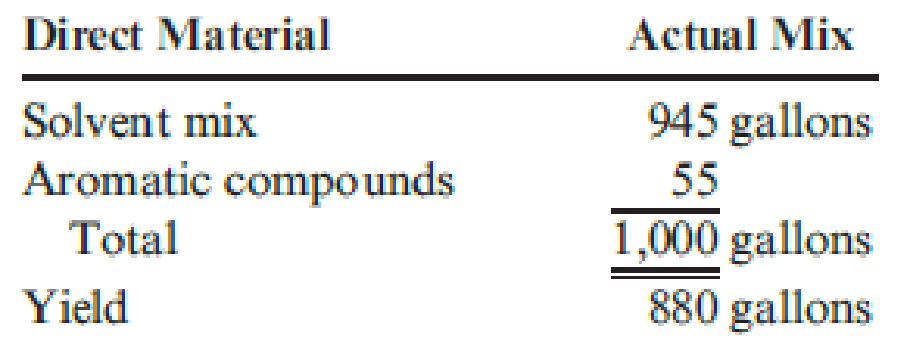# Chypre, Inc., produces a cologne mist using a solvent mix (water and pure alcohol) and aromatic compounds (the scent base) that it sells to other companies for bottling and sale to consumers. Chypre developed the following standard cost sheet: On May 2, Chypre produced a batch of 1,000 gallons with the following actual results: Required: 1. Calculate the yield ratio. 2. Calculate the standard cost per unit of the yield. (Round to the nearest cent.) 3. Calculate the direct materials yield variance. (Round to the nearest cent.) 4. Calculate the direct materials mix variance. (Round to the nearest cent.)### Cornerstones of Cost Management (C...

4th Edition
Don R. Hansen + 1 other
Publisher: Cengage Learning
ISBN: 9781305970663

#### Solutions

Chapter
Section### Cornerstones of Cost Management (C...

4th Edition
Don R. Hansen + 1 other
Publisher: Cengage Learning
ISBN: 9781305970663
Chapter 9, Problem 17E
Textbook Problem
389 views

## Chypre, Inc., produces a cologne mist using a solvent mix (water and pure alcohol) and aromatic compounds (the scent base) that it sells to other companies for bottling and sale to consumers. Chypre developed the following standard cost sheet:On May 2, Chypre produced a batch of 1,000 gallons with the following actual results:Required: 1. Calculate the yield ratio. 2. Calculate the standard cost per unit of the yield. (Round to the nearest cent.) 3. Calculate the direct materials yield variance. (Round to the nearest cent.) 4. Calculate the direct materials mix variance. (Round to the nearest cent.)

1.

To determine

Compute the yield ratio.

### Explanation of Solution

Direct material yield variance: The direct materials yield variance is calculated to show the extent to which the amount of input resulted in the estimated amount of output.

The following formula is used to calculate yield variance:

Yield variance = (Standard yieldActual yield)SPy

The following formula is used to calculate yield ratio:

Yield ratio = (Total outputTotal input)

The following formula is used to calculate standard yield:

Standard yield = Yield ratio×Total actual inputs

The following formula is used

2.

To determine

Compute the standard cost per unit of the yield.

3.

To determine

Compute the direct materials yield variance.

4.

To determine

Compute the direct materials mix variance.

### Still sussing out bartleby?

Check out a sample textbook solution.

See a sample solution

#### The Solution to Your Study Problems

Bartleby provides explanations to thousands of textbook problems written by our experts, many with advanced degrees!

Get Started

Find more solutions based on key concepts
COST OF COMMON EQUITY WITH FLOTATION Ballack Co.s common stock currently sells for 46 75 per share. The growth ...

Fundamentals of Financial Management, Concise Edition (with Thomson ONE - Business School Edition, 1 term (6 months) Printed Access Card) (MindTap Course List)

What is a nondiscretionary bonus?

PAYROLL ACCT.,2019 ED.(LL)-TEXT

What is the difference between tactical and strategic decisions?

Managerial Accounting: The Cornerstone of Business Decision-Making

List the four general control areas.

Accounting Information Systems